# Gauss sum

A trigonometric sum of the form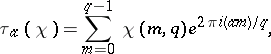where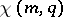is a numerical character modulo. A Gauss sum is fully defined by specifying the characterand the number. Such sums were studied by C.F. Gauss (1811) for an odd prime number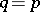and the character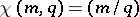, whereis the Legendre symbol. In such a case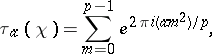(*)

where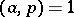. By studying the properties of the sum (*), Gauss found the exact expression for the modulus of this sum: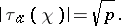He also solved the more difficult problem of determining the sign of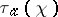, and showed that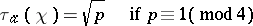and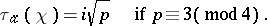Gauss utilized the properties of the sum (*) to solve certain problems in number theory; a particular case is one of the proofs of the quadratic reciprocity law.

The significance of Gauss sums in number theory only became evident in the 1920s. At that time H. Weyl used general trigonometric sums (cf. Weyl sum) in his study on uniform distribution. These sums were used at the same time by I.M. Vinogradov to obtain an estimate from above of the smallest quadratic non-residue modulo.

With the aid of Gauss sums it is possible to establish a relation between two important objects in number theory — viz. between the multiplicative charactersand the additive characters(only the case of a prime moduleis considered, for the sake of simplicity). The setof all complex-valued functionswith periodforms a-dimensional vector space over the field of complex numbers. If one defines the scalar product inby putting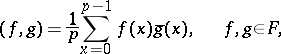then the functions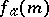,, form an orthonormal basis of; here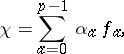where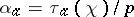. Thus, the Gauss sums(up to the factor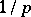) are coordinates in the expansion of the multiplicative characterby the additive characters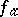. The possibility of a linear representation of any character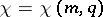as a linear combination of exponents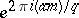, which follows from the properties of Gauss sums of general type, forms the basis of the proof of the functional equation for the-function.

These ideas are effectively applied in the method of the large sieve, and in passing from an estimate of sums of additive characters to an estimate of sums of multiplicative characters. Gauss sums are also employed to represent-functions by finite sums. Such a representation is employed in the problem of the number of divisor classes of a cyclotomic field.

The problem of the sign of the Gauss sumbelonging to a quadratic character may be stated in a more general manner for a Gauss sum belonging to a characterof order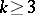. The Kummer hypothesis on cubic Gauss sums with a prime module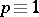(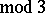), as well as its generalization to, arises.